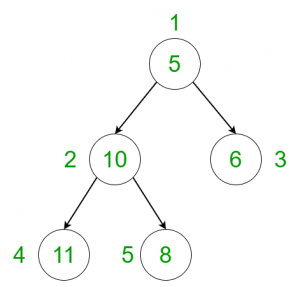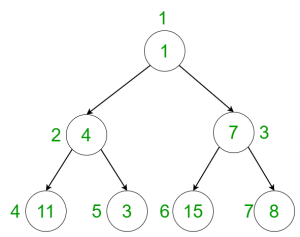Related Articles

# Count nodes in the given tree whose weight is a fibonacci number

• Last Updated : 23 Jun, 2021

Given a tree with the weights of all the nodes, the task is to count the number of nodes whose weight is a Fibonacci number.
Examples:

Input:Output:
Explanation:
Nodes having weights 5 and 8 are fibonacci nodes.
Input:Output:
Explanation:
Nodes having weights 1, 3 and 8 are fibonacci nodes.

Approach: The idea is to perform a dfs on the tree and for every node, check whether the weight is a Fibonacci number or not.

1. Generate a hash containing all the Fibonacci numbers using Dynamic programming.
2. Using depth-first search traversal, traverse through every node of the tree and check whether the node is a Fibonacci number or not by checking if that element is present in the precomputed hash or not.
3. Finally, print the total number of Fibonacci nodes.

Below is the implementation of above approach:

## C++

 `// C++ program to count the number of nodes` `// in the tree whose weight is a` `// Fibonacci number`   `#include ` `using` `namespace` `std;`   `const` `int` `sz = 1e5;` `int` `ans = 0;`   `vector<``int``> graph;` `vector<``int``> weight(100);`   `// To store all fibonacci numbers` `set<``int``> fib;`   `// Function to generate fibonacci numbers using` `// Dynamic Programming and create hash table` `// to check Fibonacci numbers` `void` `fibonacci()` `{` `    ``// Inserting the first two Fibonacci numbers` `    ``// in the hash` `    ``int` `prev = 0, curr = 1, len = 2;` `    ``fib.insert(prev);` `    ``fib.insert(curr);`   `    ``// Computing the Fibonacci numbers until` `    ``// the maximum number and storing them` `    ``// in the hash` `    ``while` `(len <= sz) {` `        ``int` `temp = curr + prev;` `        ``fib.insert(temp);` `        ``prev = curr;` `        ``curr = temp;` `        ``len++;` `    ``}` `}`   `// Function to perform dfs` `void` `dfs(``int` `node, ``int` `parent)` `{` `    ``// Check if the weight of the node` `    ``// is a Fibonacci number or not` `    ``if` `(fib.find(weight[node]) != fib.end())` `        ``ans += 1;`   `    ``// Performing DFS to iterate the` `    ``// remaining nodes` `    ``for` `(``int` `to : graph[node]) {` `        ``if` `(to == parent)` `            ``continue``;` `        ``dfs(to, node);` `    ``}` `}`   `// Driver code` `int` `main()` `{` `    ``// Weights of the node` `    ``weight = 5;` `    ``weight = 10;` `    ``weight = 11;` `    ``weight = 8;` `    ``weight = 6;`   `    ``// Edges of the tree` `    ``graph.push_back(2);` `    ``graph.push_back(3);` `    ``graph.push_back(4);` `    ``graph.push_back(5);`   `    ``// Generate fibonacci numbers` `    ``fibonacci();`   `    ``// Call the dfs function to` `    ``// traverse through the tree` `    ``dfs(1, 1);`   `    ``cout << ans << endl;`   `    ``return` `0;` `}`

## Java

 `// Java program to count the number of nodes` `// in the tree whose weight is a` `// Fibonacci number` `import` `java.util.*;`   `class` `GFG{` ` `  `static` `int` `sz = (``int``) 1e5;` `static` `int` `ans = ``0``;` ` `  `static` `Vector []graph = ``new` `Vector[``100``];` `static` `int` `[]weight = ``new` `int``[``100``];` ` `  `// To store all fibonacci numbers` `static` `HashSet fib = ``new` `HashSet();` ` `  `// Function to generate fibonacci numbers using` `// Dynamic Programming and create hash table` `// to check Fibonacci numbers` `static` `void` `fibonacci()` `{` `    ``// Inserting the first two Fibonacci numbers` `    ``// in the hash` `    ``int` `prev = ``0``, curr = ``1``, len = ``2``;` `    ``fib.add(prev);` `    ``fib.add(curr);` ` `  `    ``// Computing the Fibonacci numbers until` `    ``// the maximum number and storing them` `    ``// in the hash` `    ``while` `(len <= sz) {` `        ``int` `temp = curr + prev;` `        ``fib.add(temp);` `        ``prev = curr;` `        ``curr = temp;` `        ``len++;` `    ``}` `}` ` `  `// Function to perform dfs` `static` `void` `dfs(``int` `node, ``int` `parent)` `{` `    ``// Check if the weight of the node` `    ``// is a Fibonacci number or not` `    ``if` `(fib.contains(weight[node]))` `        ``ans += ``1``;` ` `  `    ``// Performing DFS to iterate the` `    ``// remaining nodes` `    ``for` `(``int` `to : graph[node]) {` `        ``if` `(to == parent)` `            ``continue``;` `        ``dfs(to, node);` `    ``}` `}` ` `  `// Driver code` `public` `static` `void` `main(String[] args)` `{` `    ``for``(``int` `i = ``0``; i < ``100``; i++) {` `        ``graph[i] = ``new` `Vector();` `    ``}`   `    ``// Weights of the node` `    ``weight[``1``] = ``5``;` `    ``weight[``2``] = ``10``;` `    ``weight[``3``] = ``11``;` `    ``weight[``4``] = ``8``;` `    ``weight[``5``] = ``6``;` ` `  `    ``// Edges of the tree` `    ``graph[``1``].add(``2``);` `    ``graph[``2``].add(``3``);` `    ``graph[``2``].add(``4``);` `    ``graph[``1``].add(``5``);` ` `  `    ``// Generate fibonacci numbers` `    ``fibonacci();` ` `  `    ``// Call the dfs function to` `    ``// traverse through the tree` `    ``dfs(``1``, ``1``);` ` `  `    ``System.out.print(ans +``"\n"``);` ` `  `}` `}`   `// This code is contributed by Rajput-Ji`

## Python3

 `# Python 3 program to count the number of nodes` `# in the tree whose weight is a` `# Fibonacci number` `sz ``=` `1e5` `ans ``=` `0`   `graph ``=` `[[] ``for` `i ``in` `range``(``100``)]` `weight ``=` `[``0` `for` `i ``in` `range``(``100``)]`   `# To store all fibonacci numbers` `fib ``=` `set``()`   `# Function to generate fibonacci numbers using` `# Dynamic Programming and create hash table` `# to check Fibonacci numbers` `def` `fibonacci():`   `    ``# Inserting the first two Fibonacci numbers` `    ``# in the hash` `    ``prev ``=` `0` `    ``curr ``=` `1` `    ``len1 ``=` `2` `    ``fib.add(prev)` `    ``fib.add(curr)`   `    ``# Computing the Fibonacci numbers until` `    ``# the maximum number and storing them` `    ``# in the hash` `    ``while` `(len1 <``=` `sz):` `        ``temp ``=` `curr ``+` `prev` `        ``fib.add(temp)` `        ``prev ``=` `curr;` `        ``curr ``=` `temp;` `        ``len1 ``+``=` `1`   `# Function to perform dfs` `def` `dfs(node, parent):` `    ``global` `ans`   `    ``# Check if the weight of the node` `    ``# is a Fibonacci number or not` `    ``if` `(weight[node] ``in` `fib):` `        ``ans ``+``=` `1`   `    ``# Performing DFS to iterate the` `    ``# remaining nodes` `    ``for` `to ``in` `graph[node]:` `        ``if` `(to ``=``=` `parent):` `            ``continue` `        ``dfs(to, node)`   `# Driver code` `if` `__name__ ``=``=` `'__main__'``:` `    ``# Weights of the node` `    ``weight[``1``] ``=` `5` `    ``weight[``2``] ``=` `10` `    ``weight[``3``] ``=` `11` `    ``weight[``4``] ``=` `8` `    ``weight[``5``] ``=` `6`   `    ``# Edges of the tree` `    ``graph[``1``].append(``2``)` `    ``graph[``2``].append(``3``)` `    ``graph[``2``].append(``4``)` `    ``graph[``1``].append(``5``)`   `    ``# Generate fibonacci numbers` `    ``fibonacci()`   `    ``# Call the dfs function to` `    ``# traverse through the tree` `    ``dfs(``1``, ``1``)`   `    ``print``(ans)`   `# This code is contributed by Surendra_Gangwar`

## C#

 `// C# program to count the number of nodes` `// in the tree whose weight is a` `// Fibonacci number` `using` `System;` `using` `System.Collections.Generic;`   `public` `class` `GFG{` `  `  `static` `int` `sz = (``int``) 1e5;` `static` `int` `ans = 0;` `  `  `static` `List<``int``> []graph = ``new` `List<``int``>;` `static` `int` `[]weight = ``new` `int``;` `  `  `// To store all fibonacci numbers` `static` `HashSet<``int``> fib = ``new` `HashSet<``int``>();` `  `  `// Function to generate fibonacci numbers using` `// Dynamic Programming and create hash table` `// to check Fibonacci numbers` `static` `void` `fibonacci()` `{` `    ``// Inserting the first two Fibonacci numbers` `    ``// in the hash` `    ``int` `prev = 0, curr = 1, len = 2;` `    ``fib.Add(prev);` `    ``fib.Add(curr);` `  `  `    ``// Computing the Fibonacci numbers until` `    ``// the maximum number and storing them` `    ``// in the hash` `    ``while` `(len <= sz) {` `        ``int` `temp = curr + prev;` `        ``fib.Add(temp);` `        ``prev = curr;` `        ``curr = temp;` `        ``len++;` `    ``}` `}` `  `  `// Function to perform dfs` `static` `void` `dfs(``int` `node, ``int` `parent)` `{` `    ``// Check if the weight of the node` `    ``// is a Fibonacci number or not` `    ``if` `(fib.Contains(weight[node]))` `        ``ans += 1;` `  `  `    ``// Performing DFS to iterate the` `    ``// remaining nodes` `    ``foreach` `(``int` `to ``in` `graph[node]) {` `        ``if` `(to == parent)` `            ``continue``;` `        ``dfs(to, node);` `    ``}` `}` `  `  `// Driver code` `public` `static` `void` `Main(String[] args)` `{` `    ``for``(``int` `i = 0; i < 100; i++) {` `        ``graph[i] = ``new` `List<``int``>();` `    ``}` ` `  `    ``// Weights of the node` `    ``weight = 5;` `    ``weight = 10;` `    ``weight = 11;` `    ``weight = 8;` `    ``weight = 6;` `  `  `    ``// Edges of the tree` `    ``graph.Add(2);` `    ``graph.Add(3);` `    ``graph.Add(4);` `    ``graph.Add(5);` `  `  `    ``// Generate fibonacci numbers` `    ``fibonacci();` `  `  `    ``// Call the dfs function to` `    ``// traverse through the tree` `    ``dfs(1, 1);` `  `  `    ``Console.Write(ans +``"\n"``);` `  `  `}` `}` `// This code contributed by Rajput-Ji`

## Javascript

 ``

Output:

`2`

Complexity Analysis:

• Time Complexity : O(N).
In dfs, every node of the tree is processed once and hence the complexity due to the dfs is O(N) if there are total N nodes in the tree. Also, for processing each node the fibonacci() function is used which has a complexity of O(N) too but since this function is executed only once, it does not affect the overall time complexity. Therefore, the time complexity is O(N).
• Auxiliary Space : O(N).
Extra space is used for the fibonacci hashset, so the space complexity is O(N).

Attention reader! Don’t stop learning now. Get hold of all the important DSA concepts with the DSA Self Paced Course at a student-friendly price and become industry ready.  To complete your preparation from learning a language to DS Algo and many more,  please refer Complete Interview Preparation Course.

In case you wish to attend live classes with experts, please refer DSA Live Classes for Working Professionals and Competitive Programming Live for Students.

My Personal Notes arrow_drop_up
Recommended Articles
Page :